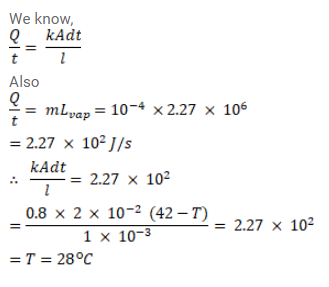# A pitcher with 1mm thick porous walls contains 10kg of water.Question:

A pitcher with $1 \mathrm{~mm}$ thick porous walls contains $10 \mathrm{~kg}$ of water. Water comes to its outer surface and evaporates at the rate of $0.1 \mathrm{~g} / \mathrm{s}$. The surface area of the pitcher (one side) $=200 \mathrm{~cm}^{2}$ The room temperature $=42^{\circ} \mathrm{C}$, latent heat of vaporization $=2.27 \times 10^{6} \mathrm{~J} / \mathrm{kg}$ and the thermal conductivity of the porous walls $=0.80 \mathrm{~J} / \mathrm{m}-\mathrm{s}-{ }^{\circ} \mathrm{C}$ Calculate the temperature of water in the pitcher when it attains a constant value.

Solution: